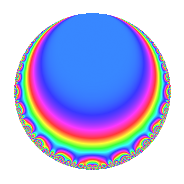# Properties

 Label 4003.2.a.aLevel 4003 Weight 2 Character orbit 4003.a Self dual yes Analytic conductor 31.964 Analytic rank 1 Dimension 2 CM no Inner twists 1

# Related objects

## Newspace parameters

 Level: $$N$$ = $$4003$$ Weight: $$k$$ = $$2$$ Character orbit: $$[\chi]$$ = 4003.a (trivial)

## Newform invariants

 Self dual: yes Analytic conductor: $$31.9641159291$$ Analytic rank: $$1$$ Dimension: $$2$$ Coefficient field: $$\Q(\sqrt{2})$$ Coefficient ring: $$\Z[a_1, a_2]$$ Coefficient ring index: $$1$$ Twist minimal: yes Fricke sign: $$1$$ Sato-Tate group: $\mathrm{SU}(2)$

## $q$-expansion

Coefficients of the $$q$$-expansion are expressed in terms of $$\beta = \sqrt{2}$$. We also show the integral $$q$$-expansion of the trace form.

 $$f(q)$$ $$=$$ $$q + \beta q^{2} + \beta q^{3} + \beta q^{5} + 2 q^{6} - q^{7} -2 \beta q^{8} - q^{9} +O(q^{10})$$ $$q + \beta q^{2} + \beta q^{3} + \beta q^{5} + 2 q^{6} - q^{7} -2 \beta q^{8} - q^{9} + 2 q^{10} + ( -2 - 2 \beta ) q^{11} + ( 4 + 2 \beta ) q^{13} -\beta q^{14} + 2 q^{15} -4 q^{16} + ( -1 - 4 \beta ) q^{17} -\beta q^{18} + ( 1 + 4 \beta ) q^{19} -\beta q^{21} + ( -4 - 2 \beta ) q^{22} + ( -6 + 2 \beta ) q^{23} -4 q^{24} -3 q^{25} + ( 4 + 4 \beta ) q^{26} -4 \beta q^{27} + ( -4 - 2 \beta ) q^{29} + 2 \beta q^{30} + ( 2 - 6 \beta ) q^{31} + ( -4 - 2 \beta ) q^{33} + ( -8 - \beta ) q^{34} -\beta q^{35} + ( -7 - 2 \beta ) q^{37} + ( 8 + \beta ) q^{38} + ( 4 + 4 \beta ) q^{39} -4 q^{40} + ( 8 + 2 \beta ) q^{41} -2 q^{42} -\beta q^{45} + ( 4 - 6 \beta ) q^{46} + ( 4 + 5 \beta ) q^{47} -4 \beta q^{48} -6 q^{49} -3 \beta q^{50} + ( -8 - \beta ) q^{51} + ( -2 - 8 \beta ) q^{53} -8 q^{54} + ( -4 - 2 \beta ) q^{55} + 2 \beta q^{56} + ( 8 + \beta ) q^{57} + ( -4 - 4 \beta ) q^{58} + ( -6 + 2 \beta ) q^{59} + ( 10 + 2 \beta ) q^{61} + ( -12 + 2 \beta ) q^{62} + q^{63} + 8 q^{64} + ( 4 + 4 \beta ) q^{65} + ( -4 - 4 \beta ) q^{66} + ( 7 - 4 \beta ) q^{67} + ( 4 - 6 \beta ) q^{69} -2 q^{70} + 6 q^{71} + 2 \beta q^{72} -3 q^{73} + ( -4 - 7 \beta ) q^{74} -3 \beta q^{75} + ( 2 + 2 \beta ) q^{77} + ( 8 + 4 \beta ) q^{78} + ( 3 - 6 \beta ) q^{79} -4 \beta q^{80} -5 q^{81} + ( 4 + 8 \beta ) q^{82} + ( 3 - 10 \beta ) q^{83} + ( -8 - \beta ) q^{85} + ( -4 - 4 \beta ) q^{87} + ( 8 + 4 \beta ) q^{88} + ( -9 + 2 \beta ) q^{89} -2 q^{90} + ( -4 - 2 \beta ) q^{91} + ( -12 + 2 \beta ) q^{93} + ( 10 + 4 \beta ) q^{94} + ( 8 + \beta ) q^{95} + ( 6 + 3 \beta ) q^{97} -6 \beta q^{98} + ( 2 + 2 \beta ) q^{99} +O(q^{100})$$ $$\operatorname{Tr}(f)(q)$$ $$=$$ $$2q + 4q^{6} - 2q^{7} - 2q^{9} + O(q^{10})$$ $$2q + 4q^{6} - 2q^{7} - 2q^{9} + 4q^{10} - 4q^{11} + 8q^{13} + 4q^{15} - 8q^{16} - 2q^{17} + 2q^{19} - 8q^{22} - 12q^{23} - 8q^{24} - 6q^{25} + 8q^{26} - 8q^{29} + 4q^{31} - 8q^{33} - 16q^{34} - 14q^{37} + 16q^{38} + 8q^{39} - 8q^{40} + 16q^{41} - 4q^{42} + 8q^{46} + 8q^{47} - 12q^{49} - 16q^{51} - 4q^{53} - 16q^{54} - 8q^{55} + 16q^{57} - 8q^{58} - 12q^{59} + 20q^{61} - 24q^{62} + 2q^{63} + 16q^{64} + 8q^{65} - 8q^{66} + 14q^{67} + 8q^{69} - 4q^{70} + 12q^{71} - 6q^{73} - 8q^{74} + 4q^{77} + 16q^{78} + 6q^{79} - 10q^{81} + 8q^{82} + 6q^{83} - 16q^{85} - 8q^{87} + 16q^{88} - 18q^{89} - 4q^{90} - 8q^{91} - 24q^{93} + 20q^{94} + 16q^{95} + 12q^{97} + 4q^{99} + O(q^{100})$$

## Embeddings

For each embedding $$\iota_m$$ of the coefficient field, the values $$\iota_m(a_n)$$ are shown below.

For more information on an embedded modular form you can click on its label.

Label $$\iota_m(\nu)$$ $$a_{2}$$ $$a_{3}$$ $$a_{4}$$ $$a_{5}$$ $$a_{6}$$ $$a_{7}$$ $$a_{8}$$ $$a_{9}$$ $$a_{10}$$
1.1
 −1.41421 1.41421
−1.41421 −1.41421 0 −1.41421 2.00000 −1.00000 2.82843 −1.00000 2.00000
1.2 1.41421 1.41421 0 1.41421 2.00000 −1.00000 −2.82843 −1.00000 2.00000
 $$n$$: e.g. 2-40 or 990-1000 Significant digits: Format: Complex embeddings Normalized embeddings Satake parameters Satake angles

## Inner twists

This newform does not admit any (nontrivial) inner twists.

## Twists

By twisting character orbit
Char Parity Ord Mult Type Twist Min Dim
1.a even 1 1 trivial 4003.2.a.a 2

By twisted newform orbit
Twist Min Dim Char Parity Ord Mult Type
4003.2.a.a 2 1.a even 1 1 trivial

## Atkin-Lehner signs

$$p$$ Sign
$$4003$$ $$1$$

## Hecke kernels

This newform subspace can be constructed as the kernel of the linear operator $$T_{2}^{2} - 2$$ acting on $$S_{2}^{\mathrm{new}}(\Gamma_0(4003))$$.